Logiciel libre de géométrie, d'analyse et de simulation multiplateforme par Yves Biton

AccueilExemples

# Use of LaTeX code : Example 1 : LaTeX display creation

publication dimanche 13 février 2011.

In this example we will demonstrate how to display dynamical LaTeX code in a MathGraph32 figure.

Create a new Figure with menu item File - New file with - Frame without vectors and choose an orthonormal frame.

With menu item Options - Current figure, tab Angle unity make sure the figure uses radian for angle unity

Use icon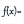to create a function f with formula f(t) = sin(t^2) + 1. Let the chekbox Draw curve selected and ask for 500 points. The function curve appears.

Use icon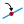to create a point linked to the x-axis and name this pointa (icon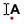).

In the same way create a point linked to the y-axis named b.

Use icon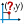to create the measure of the abscissa of point a (click on the point. This abscissa is represented as xCoord(a,O,I,J)).

In the same way create the measure of the abscissa of point b.

Use icon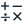to create a calculus named a with formula xCoord(a,O,I,J) (you can use button Values).

In the same way create a calculus named b with formula xCoord(b,O,I,J) using button Values.

In the color palette, set the color blue activated.

Use icon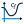to create the curve of function f on the interval [a ; b]. For this fill in the dialog bow as shown underneath.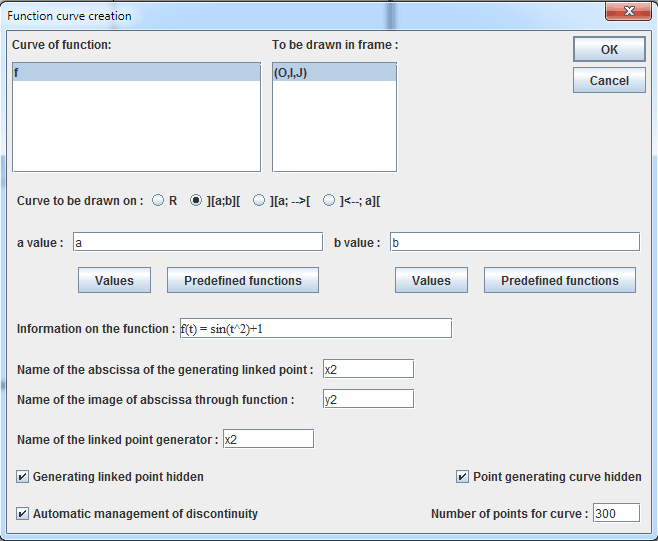We will now fill the surface between the curve ane the x-axis on interval [a ; b]. For this click on icon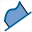(creation of surface delimited by a point locus and a line). Click on the blue curve (you will be asked for the first or last object : choose last) then on the x-axis. The surface appears.

Now let’s calculate an approximated value of the integral.
For this create a new calculus with icon. For name enter I and enter formula integral(f(t),t,a,b).
You can use button Predefined function for help on the syntax.

Let’s now create a LaTeX display. For this click on icon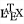the click on a free spot in the bottom left corner of the figure.

A dialog box pops up. Click on icon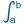.

The LaTeX code for an integral is inserted as show underneath. The caret position is set inside the first pair of brackets for the lower bound of the integral.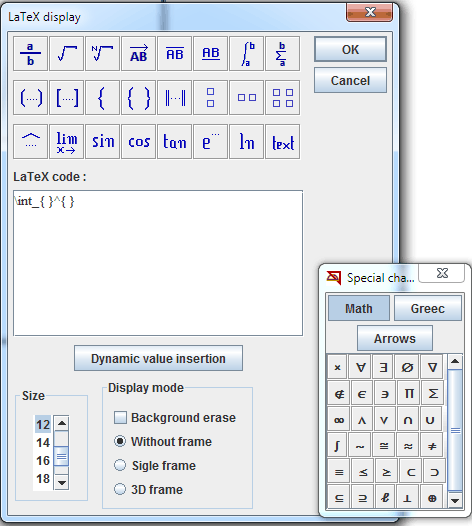We will display here a dynamical display of the value of a. For this click on button Dynamic value insertion.

In the list of values, click on a then click on button Insert code.

Le code LaTex est maintenant devenu  \int_{\Val{a} }^{ } .

Now set the caret position inside the second bracket (you can use Tab key) and use button Dynamic value insertion to insert a dynamical display of b value.

The LaTeX code is now  \int_{\Val{a} }^{\Val{b} }  .

Complete the code as follows :

 \int_{\Val{a} }^{\Val{b} } f(t)dt =

then use button Dynamic value insertion to insert a dynamical display of I with 6 digits.

the LaTeX code is now :

 \int_{\Val{a} }^{\Val{b} } f(t)dt = \Val{I,6}

In the Size list select 14 and validate.

If you wand to modify this LaTeX display,you just have to click on icon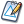and then click on the display.

You can see underneath the figure animated via MathGraph32 applet :

You can capture points a and b to visualize the fact that the LaTeX display is dynamical.